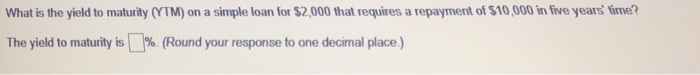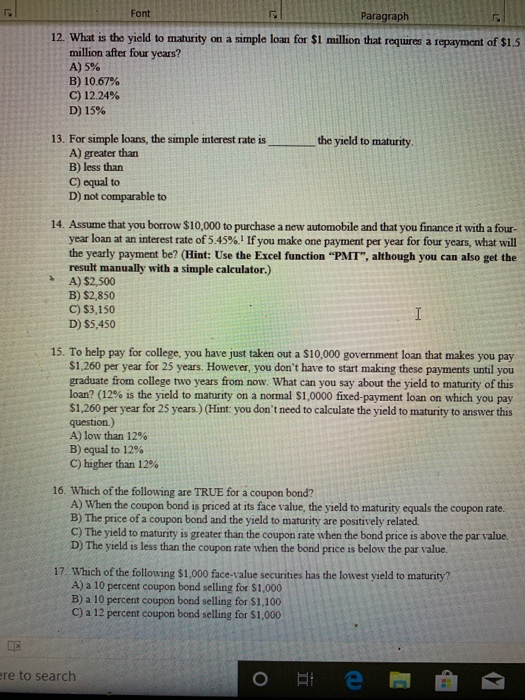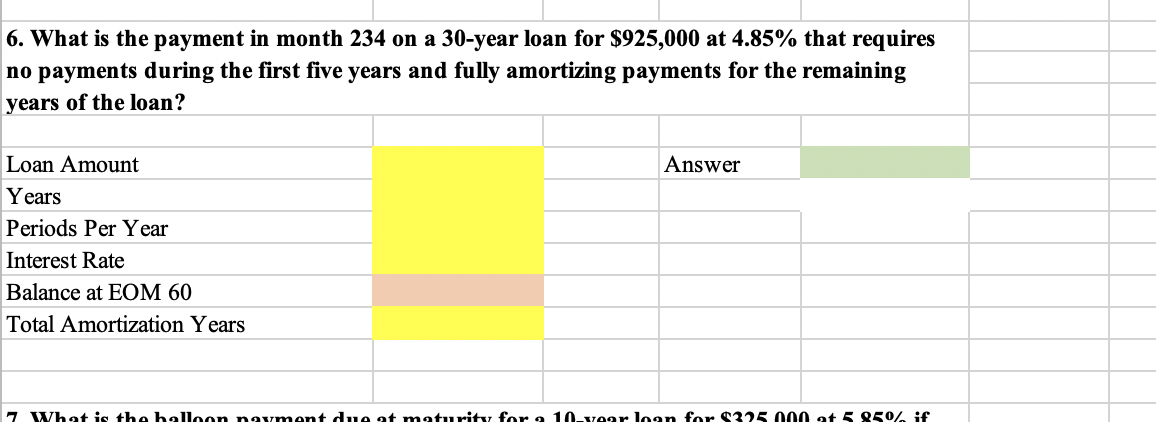Question

# calculate the yield to maturity on a loan for a \$12,000 that requires month payments of...

calculate the yield to maturity on a loan for a \$12,000 that requires month payments of \$127.28 for 10 years.

**please show how you get this**

You can calculate it through I/Y function on a financial calculator

Insert N = 10 x 12 = 120, PMT = 127.28, PV = -12,000, FV = 0

=> Compute I/Y = 0.42% (monthly)

Annualized YTM = 0.42% x 12 = 5.00%

#### Earn Coins

Coins can be redeemed for fabulous gifts.

Similar Homework Help Questions
• ### Please show all work Yield to maturity. (a) Find the yield to maturity on a 1-month...

Please show all work Yield to maturity. (a) Find the yield to maturity on a 1-month T-bill with \$100,000 face value that sold for a price of \$99,667.77. Answer: i = 0.04 (b) Consider a loan of \$400,000 to be repaid with annual payments over 25 years. Find the fixed payments that would be required for the yield to maturity on the loan to be 6%. Answer: FP = \$31,290.68. (c) Consider a loan of \$200,000 to be repaid with...

• ### What is the yield to maturity (YTM) on a simple loan for \$2,000 that requires a...What is the yield to maturity (YTM) on a simple loan for \$2,000 that requires a repayment of \$10,000 in five years time? The yield to maturity is % (Round your response to one decimal place.

• ### Font Paragraph 12. What is the yield to maturity on a simple loan for \$1 million...Font Paragraph 12. What is the yield to maturity on a simple loan for \$1 million that requires a repayment of \$1.5 million after four years? A) 5% B) 10.67% C) 12 24% D) 15% the yield to maturity. 13. For simple loans, the simple interest rate is_ A) greater than B) less than C) equal to D) not comparable to 14. Assume that you borrow \$10,000 to purchase a new automobile and that you finance it with a four-...

• ### A \$12,000 loan is repaid by semiannual payments of \$1500 each. Interest on the loan is...

A \$12,000 loan is repaid by semiannual payments of \$1500 each. Interest on the loan is 10% compounded semiannually. How long will it take to pay off the loan? Select one: a. 10 years b. 10.4 years c. 5 years d. 21 years e. 5.2 years

• ### Your car loan requires payments of \$300 a month for the first year and payments of...

Your car loan requires payments of \$300 a month for the first year and payments of \$500 per month the second year. The annual interest rate is 6%, and payments begin in one month. What is the present value of this two-year loan?

• ### problem 5-13 yield to maturity and current yield

you just purchased a bond that matures in 5 years. The bond has a face value of \$1000. and has an 8% annual coupon. The bond has a current yield rate of 8.21 precent.What is the bonds yield to maturity.please show all your work on how you get to the answer please. The answer is not enough for me to understand how to get the answer.

• ### SHOW WORK PLEASE!!!!!!! 1. Complete problem: Yield to Maturity for Annual Payments XYZ Corporation’s bonds have...

SHOW WORK PLEASE!!!!!!! 1. Complete problem: Yield to Maturity for Annual Payments XYZ Corporation’s bonds have 14 years remaining to maturity. Interest is paid annually, the bonds have a \$1,000 par value, and the coupon interest rate is 10%. The bonds sell at a price of \$950. What is their yield to maturity? Show your work.

• ### Please use excel functions 6. What is the payment in month 234 on a 30-year loan...Please use excel functions 6. What is the payment in month 234 on a 30-year loan for \$925,000 at 4.85% that requires no payments during the first five years and fully amortizing payments for the remaining years of the loan? Answer Loan Amount Years Periods Per Year Interest Rate Balance at EOM 60 Total Amortization Years 7 What is the balloon nayment du at maturity for a 10-year loan for \$325 000 at 5 9507 if

• ### Calculate yield to maturity for a bond that is trading at \$890 carrying a coupon rate...

Calculate yield to maturity for a bond that is trading at \$890 carrying a coupon rate of 7.5 percent with 10 years left to maturity. Please show with ti-83

• ### Problem 1: Using a Spreadsheet to Calculate Yield to Maturity What is the yield to maturity...

Problem 1: Using a Spreadsheet to Calculate Yield to Maturity What is the yield to maturity on the following bonds; all have a maturity of 10 years and a face value of \$1,000, and a coupon rate of 9 percent (paid semiannually). The bonds' current market values are \$945.50, \$987.50, \$1,090.00, and \$1,225.875, respectively. Market Total Coupon Periodic Coupon Face SEMI-ANNUAL => Yield to Value (PV) Payments (NPER) Rate (ANNUAL) Payment (PMT) Value (FV) YTM (RATE) Maturity \$945.50 20 9%...